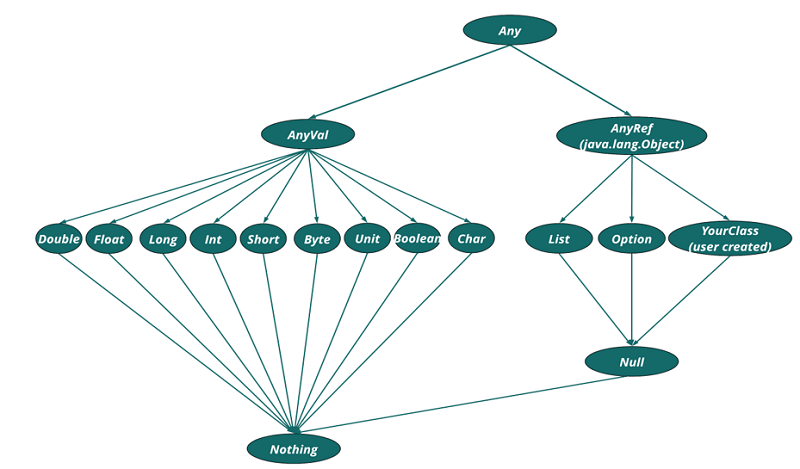# Scala Type Hierarchy

In this tutorial, we will learn about the type hierarchy in Scala and examples of a hierarchy. By Shivang Yadav Last updated : April 02, 2023

## Type Hierarchy

In Scala programming language everything is an object. Even data types are treated as objects in Scala and have some method that performs operations of the data stored by them. So, all the data types, functions/methods, classes you define have a type and the type is defined in the type hierarchy.

## Type Hierarchy diagram

Let's see the type hierarchy diagram that will help you understand the type hierarchy.Now, let's see all the main types of the type hierarchy.

## Types of the type hierarchy

### 1) Any

The parent type of all the types in Scala is Any type. It called the top type and has some universal method that can be used by all types in the type hierarchy.

#### Example of display the use of any type

```object MyClass {
def main(args: Array[String]): Unit = {
println("Creating a set of type = 'Any' ")
val anySet: Set[Any] = Set(34, "includehelp", true, List(32, 3, 45))
println("Set is : " + anySet)
}
}
```

#### Output

```Creating a set of type = 'Any'
Set is : Set(34, includehelp, true, List(32, 3, 45))
```

The Any type has two direct subtypes: AnyVal and AnyRef types.

### 2) AnyVal

The child type of Any that represents value type is AnyVal. In scala, there are in total nine predefined value types and these are sub-type of the AnyVal. They are :

• Double represents a double-precision decimal type.
• Float represents a single-precision decimal type.
• Long represents 64 bit signed integer type(value: -9223372036854775808 to 9223372036854775807).
• Int represents 32 bit signed integer type (value: -2147483648 to 2147483647).
• Short represents 16 bit signed integer type (value: -32768 to 32767).
• Byte represents 8 bit signed value (value: -128 to 127).
• Char represents character type (value: all valid character).
• Unit represents a unit type.
• Boolean represents boolean type (value: true/false).

#### Example of display the use of AnyVal

```object MyClass {
def main(args: Array[String]): Unit = {
println("Creating a set of type = 'AnyVal' ")
val anySet: Set[AnyVal] = Set(987.12331, 89.76, 87694421, 34, 'i', true)
println("Set is : " + anySet)
}
}
```

#### Output

```Creating a set of type = 'AnyVal'
Set is : HashSet(89.76, 87694421, true, i, 34, 987.12331)
```

### 3) AnyRef

The child type of Any that represents non-value type is AnyRef. This type is referenced by all user-defined classes by default.

#### Example of display the use of AnyRef

```object MyClass {
def main(args: Array[String]): Unit = {
println("Creating a set of type = 'AnyRef' ")
val anySet: Set[AnyRef] = Set("includehelp")
println("Set is : " + anySet)
}
}
```

#### Output

```Creating a set of type = 'AnyRef'
Set is : Set(includehelp)
```

These were about the main type of Scala library that provides some basic functionality to all entities in the Scala.

There are two important types that are at the bottom of the hierarchy. They are,

1. Nothing
2. Null

### a) Nothing

The type at the bottom of the hierarchy that is a subtype of all the types in Scala is Nothing. There is no value of this type.

#### Example of display the use of Nothing

```object MyClass {
def main(args: Array[String]): Unit = {
println("Creating a set of type = 'Nothing' ")
val anySet: Set[Nothing] = Set()
println("Set is : " + anySet)
}
}
```

#### Output

```Creating a set of type = 'Nothing'
Set is : Set()
```

The Nothing type in Scala is used to signal non-termination like program exit.

### b) Null

The type is subtype of reference types (for all subtypes of AnyRef type).

#### Example of display the use of Null

```object MyClass {
def main(args: Array[String]): Unit = {
println("Creating a set of type = 'Null' ")
val anyRef: List[Null] = List()
println("Set is : " + anyRef)
}
}
```

#### Output

```Creating a set of type = 'Null'
Set is : List()
```